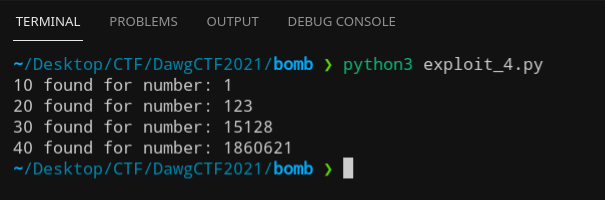# Phase 4 (150 pts)

Double the points of the previous challenge!

## Problem Statement#

``````This is the phase you have been waiting for... one may say it's the golden stage!
Let's switch things up! Numerical inputs map to line numbers in rockyou.txt, and each word isseparated by a '_' (if the phase's solution is 4 5, the flag would be DawgCTF{password_iloveyou})
Author: treap_treap``````

## Solution#

As usual, Ghidra to the rescue.

### Ghidra#

``````phase4(undefined8 param_1,undefined4 param_2,undefined4 param_3,undefined4 param_4,      undefined4 param_5,undefined4 param_6,undefined4 param_7,undefined4 param_8,char *param_9)
{  long lVar1;  void *__ptr;  long output;  long in_FS_OFFSET;  undefined4 extraout_XMM0_Da;  undefined4 result;  int counter;  long array ;  long local_20;  long curr;
local_20 = *(long *)(in_FS_OFFSET + 0x28);  puts("\nThis is the phase you have been waiting for... one may say it\'s the golden stage!");  puts(      "Let\'s switch things up! Numerical inputs map to line numbers in rockyou.txt, and each word is separated by a \'_\' (if the phase\'s solution is 4 5, the flag would be DawgCTF{password_iloveyou})"      );  result = 1;  array = 1;  array = 0x7b;  array = 0x3b18;  array = 0x1c640d;  lVar1 = func4(10);  __ptr = calloc(4,4);  getInput(extraout_XMM0_Da,param_2,param_3,param_4,param_5,param_6,param_7,param_8,4,param_9,           "%d%d%d%d",__ptr,(long)__ptr + 4,(long)__ptr + 8,(char)__ptr + '\f');  counter = 0;  while (counter < 4) {    curr = array[counter];    output = func4(*(int *)((long)__ptr + (long)counter * 4));    if (curr * (int)lVar1 - output != 0) {      result = 0;    }    counter = counter + 1;  }  free(__ptr);  if (local_20 != *(long *)(in_FS_OFFSET + 0x28)) {                    /* WARNING: Subroutine does not return */    __stack_chk_fail();  }  return result;}``````

### Solve.py#

Essentially, `func4` is just a glorified `fibonacci number creator`. We can then implement it in our python code to solve it.

``````a = [1, 0x7b, 0x3b18, 0x1c640d]
def func(n):    a = 0    b = 1    if n < 1:        return 0    elif n == 1:        return 1    else:        for i in range(2,n + 1):            c = a + b            a = b            b = c        return b
e = func(10)for n in a:    for i in range(1000):        z = n * e - func(i)        if z == 0:            print(str(i) + " found for number: " + str(n))            break``````

### Output#### Flag#

`DawgCTF{abc123_qwerty_anthony_123123}`